Home | | Electronic Circuits I | Hybrid - π equivalent circuits of BJTs

# Hybrid - π equivalent circuits of BJTs

At low frequencies, we can analyze the transistor using h-parameters.

Hybrid - π equivalent circuits of BJTs:

At low frequencies, we can analyze the transistor using h-parameters. But for high frequency, analysis of h-parameter model is not suitable for following reasons.

1.     The values of h-parameters are not constant at high frequencies. So it is necessary to analyze transistor at each and every frequency which is impractical.

2.     At high frequency h-parameters become complex in nature.

Due to the above reasons, modified T model and hybrid ∏ models are used for high frequency analysis of the transistor. These models give a reasonable compromise between accuracy and simplicity to do high frequency analysis of the transistor.

Hybrid - π common emitter transistor model:

Common emitter circuit is most important practical configuration and this is useful for the analysis of transistor using hybrid - ∏ model. The following figure shows the hybrid - ∏ model for a transistor in CE configuration. For this model, all parameters are assumed to be independent of frequency. But they may vary with the quiescent operating point.Elements in hybrid – π model:

Cb’e and Cb’c :Forward biased PN junction exhibits a capacitive effect called diffusioncapacitance. This capacitive effect of normally forward biased base- emitter junction of the transistor is represented by Cb’e or Ce. The diffusion capacitance is connected between b’ and e represents the excess minority carrier storage in the base.

The reverse bias PN junction exhibits a capacitive effect called transition capacitance. This capacitive effect of normally reverse biased collector base junction of the transistor is represented by Cb’c or Cc.

rbb’:The internal node b’ is physically not accessible bulk node b represents external baseterminal.

rb’eIt is the portion of the base emitter which may be thought of as being in series withthe collector junction. This establishes a virtual base b’ for junction capacitances to be connected instead of b.

rb’cDue to early effect, varying voltages across collector to emitter junction results inbase-width modulation. A change in the effective base -width causes the emitter current to change. This feedback effect between output and input is taken into account by connectinggb’c or rb’c between b’ and c.

gmDue to small changes in voltage Vb’eacross emitter junction, there is excess minoritycarrier concentration injected into the base which is proportional to Vb’e. So resulting small signal collector current with collector shorted to the emitter is also proportional to Vb’e.

gm is also called as transconductance and it is given as,rceIt is the output resistance. It is also the result of early effect.

Hybrid – π parameter values:

The following table shows the typical values for hybrid - π parameters at room temperature and for Ic = 1.3mA.Hybrid – π conductances:

Transistor Transconductancegm:

Let us consider a p-n-p transistor in CE configuration with Vcc bias in the collector circuit as shown in the above figure.

Transconductance gm is given as,The collector current in active region is given as,For Ic = 1.3mA, gm = 0.05mho or 50 mA/V. For Ic = 7.8mA, gm = 0.3mho or 300mA/V. These values are much larger than the transconductances obtained with FETs.

Input Conductance gb’eFirst consider h-parameter model for CE configuration. Applying KCL to output circuit,Making Vce = 0, the short circuit current gain hfe is defined asFeedback Conductance gb’c:

Let us consider h-parameter model for CE configuration with input open circuit (Ib = 0), Vi is given as,Fig. Hybrid –π model for CE configuration

With Ib = 0, Vce is given as,Voltage between b’ and e, Vb’e can be given as,Substituting the value of Vi,Substituting the value of rb’e,Base Spreading Resistance rbb’:Output Resistance gce:

Using h-parameters output conductance is given as,Applying KCL to the output circuit,Relation between hybrid-π and h-parameters:Hybrid – π capacitances: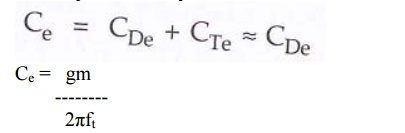Ce=  gm/2πft

CE short circuit current gain using hybrid- π model: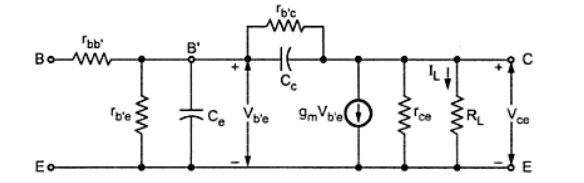Fig. Hybrid- π model for a single transistor with a resistive load RLThe current gain for the circuit is,fβ (Cutoff frequency):

It is the frequency at which the transistor short circuit CE current gain drops by 3dB or 1/√√2 times from its value at low frequency. It is given as,fα (Cut-off frequency):

It is the frequency at which the transistor short circuit CB current gain drops by 3dB or 1/√√2 times from its value at low frequency.

The current gain for CB configuration is given as,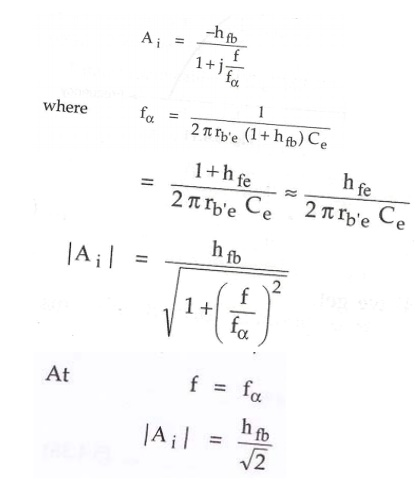Parameter fT:

It is the frequency at which short circuit CE current gain becomes unity.

At f = fT,The ratio of fT / fβ is quite large compared to 1.

fT = gm / 2πCe

Problem:

Short circuit CE current gain of transistor is 25 at a frequency of 2MHz if fβ = 200 kHz. Calculate (i) fT (ii) hfe (iii) Find |Ai| at a frequency of 10 MHz and 100 MHz.

Solution:Current gain with resistive load:For further simplification,

At output circuit value of Cc can be calculated as,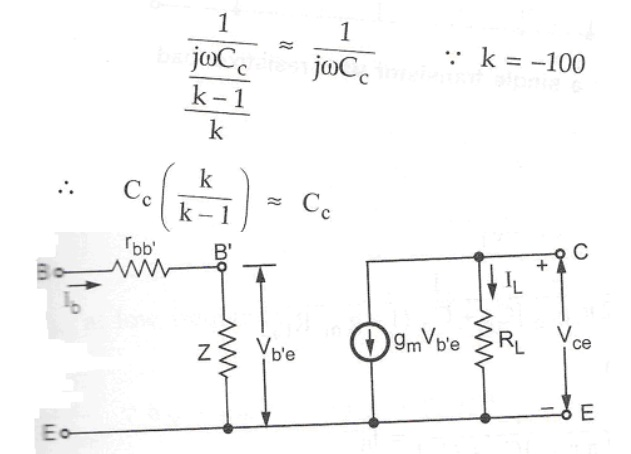Fig. Simplified hybrid – π model for CE with RL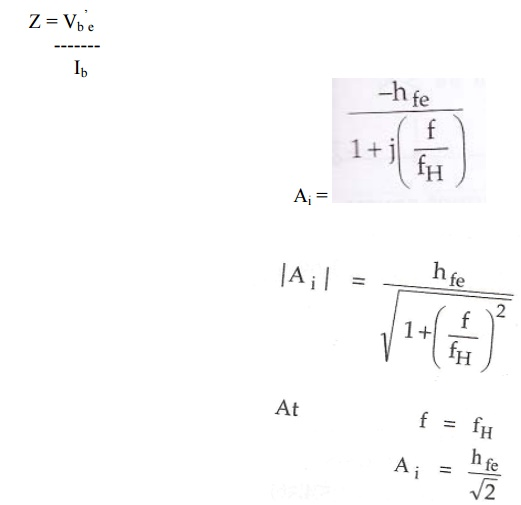fH is the frequency at which the transistor gain drops by 3dB or 1/√2 times from its value at low frequency. It is given asCurrent gain including source resistance:Voltage gain including source resistance:Cutoff frequency including source resistance:Gain Bandwidth Product:

i. Gain Bandwidth Product for Voltage:ii. Gain Bandwidth Product for current:Study Material, Lecturing Notes, Assignment, Reference, Wiki description explanation, brief detail
Electronic Circuits : Frequency Analysis of BJT and MOSFET Amplifiers : Hybrid - π equivalent circuits of BJTs |